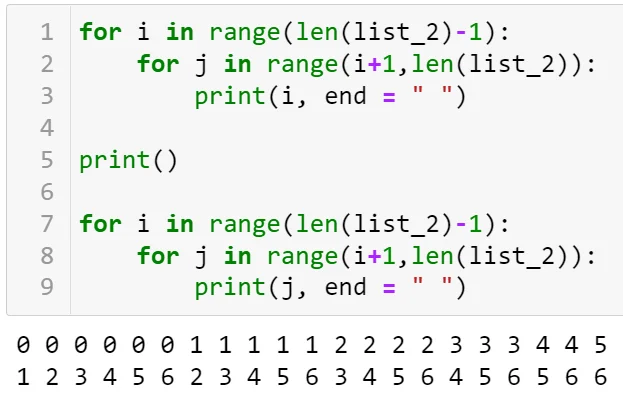09 Sept 2022

Posted on:

12 May 2022

0

# Is it ok if i use this code?

list_2 = [2,3,4,7,8,10,0]

def two_sum(listtarget):
d = {}
for i in range(len(list)-1):
for j in range(i+1,len(list)):
if (list[i] + list[j]) == target:
d[i] = j
return d

two_sum(list_2,10)

Instructor
Posted on:

13 May 2022

0

Dear Aziz,

Your solution is great! Here are some suggestion I could give.

The first one concerns the name you have given to the first parameter in the `two_sums()` function. The word `list` is a special word in Python - notice how it's colored differently than the rest of the text. Therefore, calling your parameter in such a way is not a good practice. Any other name would do - Giles has, for example, called this parameter `nums` which doesn't mean anything in Python.

Next, examine how the `range()` function behaves (in the code below, I have used your variable `list_2`):On the first row, I have displayed the index `i`, while on the second row the index `j`. Notice that, in your solution, you don't take into account the case where a number, added with itself, gives the target number. For example, changing `list_2` to `[5, 3, 4, 7, 8, 10, 0]` (I have changed the 2 at the beginning to a 5) won't give `0:0` as a possible solution. I would therefore change the second `for`-loop to:

``````for j in range(i,len(nums)):
``````

Following the same logic, if we have `list_2` being of the form `[2, 3, 4, 7, 8, 10, 5]` (I have changed the 0 at the end to a 5), we won't have `6:6` as a possible solution. I would therefore change the first `for`-loop as follows:

``````for i in range(len(nums)):
``````

To sum up, the code that I suggest is the following:

``````def two_sum(nums, target):
d = {}
for i in range(len(nums)):
for j in range(i,len(nums)):
if (nums[i] + nums[j]) == target:
d[i] = j
print(d)
return d
``````

I have also added a line of code that prints out the dictionary every time a new element is added to it. In this way, you can track the progress and examine the behavior of the code.

Hope this answer helps! Keep up the good work!

Kind regards,
365 Hristina

Posted on:

14 May 2022

0

Thank you very much )

Super learner
Posted on:

09 Sept 2022

0

def two_sum(nums, target):
d={}
count = 0
for i in range(len(nums)):
for j in range(i,len(nums)):
if nums[i] + nums[j] == target:
count=count + 1
d={nums[i], nums[j]}
print(d)
if count != 0 :
return 'two sums exists'
else :
return('two-sums does Not exist')